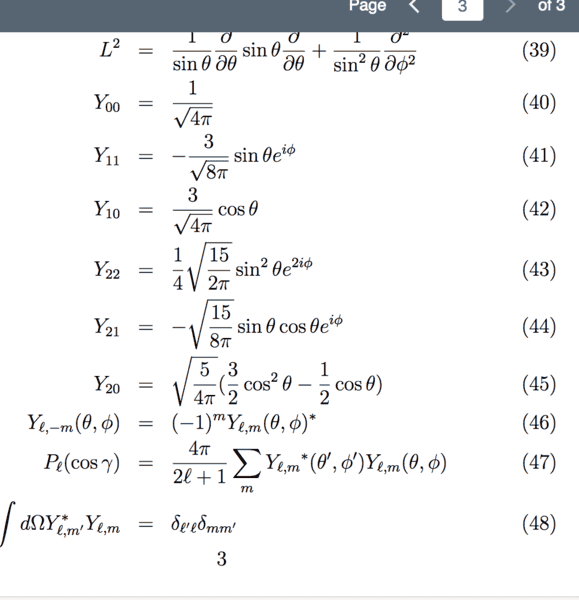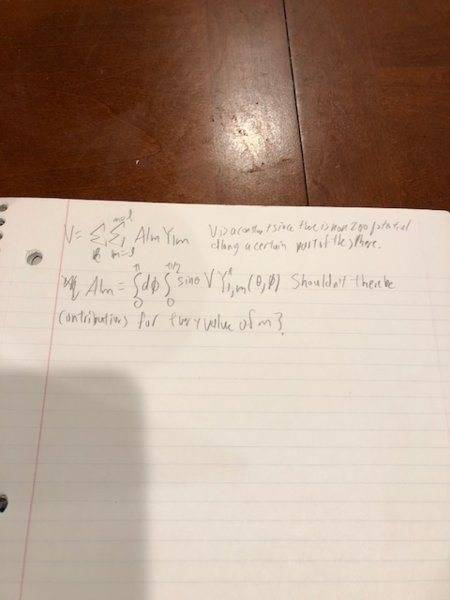# Spherical Harmonics

## Homework Statement

A sphere of radius a has V = 0 everywhere except between 0 < θ < π/2 and 0 < φ < π. Write an expression in spherical harmonics for the potential for r > a. For which values of m are there contributions? Determine the contributions through l= 2. How could you determine the leading contribution using just superposition?

## Homework Equations

Again I will be uploading images. I am only looking for answer in terms of a general formula. Not a fully drawn out calculation.## The Attempt at a Solution

I will be uploading a solution. Again the solution they are looking for is very general. What I am confused about is that this problem implies that for certain values of m there are no contributions but that does not seem to be the case with these bounds of integration. Also I do not understand the superposition part of this question.#### Attachments

Gold Member
Look at the symmetry of the problem and compare it with the symmetry of the spherical harmonics.

Look at the symmetry of the problem and compare it with the symmetry of the spherical harmonics.
I’m integrating all the phi terms they don’t seem to go to 0.

Gold Member
I’m integrating all the phi terms they don’t seem to go to 0.

That's why you don't just throw math a problem that involves physics. It appears your potential independent of \phi, correct? If so,which m values should you not get zero answers for? Second, you were asked how you could guess a term or terms by superposition. Look at the symmetry of the Y_lm you can have after figuring out which ones those are (that you got by symmetry). You have the potential on a sphere which is V on the upper hemisphere and 0 on the lower hemisphere. It might help to shift the potential by -V/2 so that the symmetry is more obvious. Then you have a constant V/2 on the entire sphere and term and and a tem with +V/2 on the top hemisphere and -V/2 on the bottom hemisphere. When you superpose them you get V on the top half and zero on the bottom half. First thing you should always do in any problem is shift the origin or shift something to make as much symmetry explicit as possible. Then when you are done, you can shift back.

Staff Emeritus
•